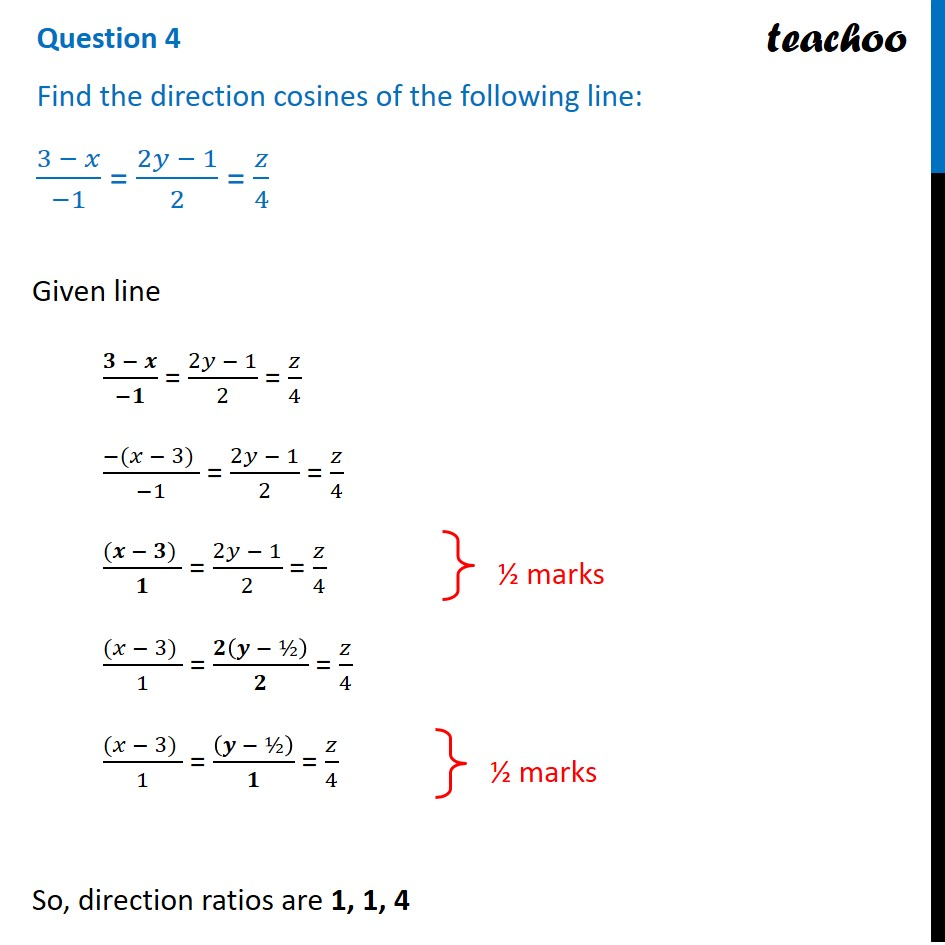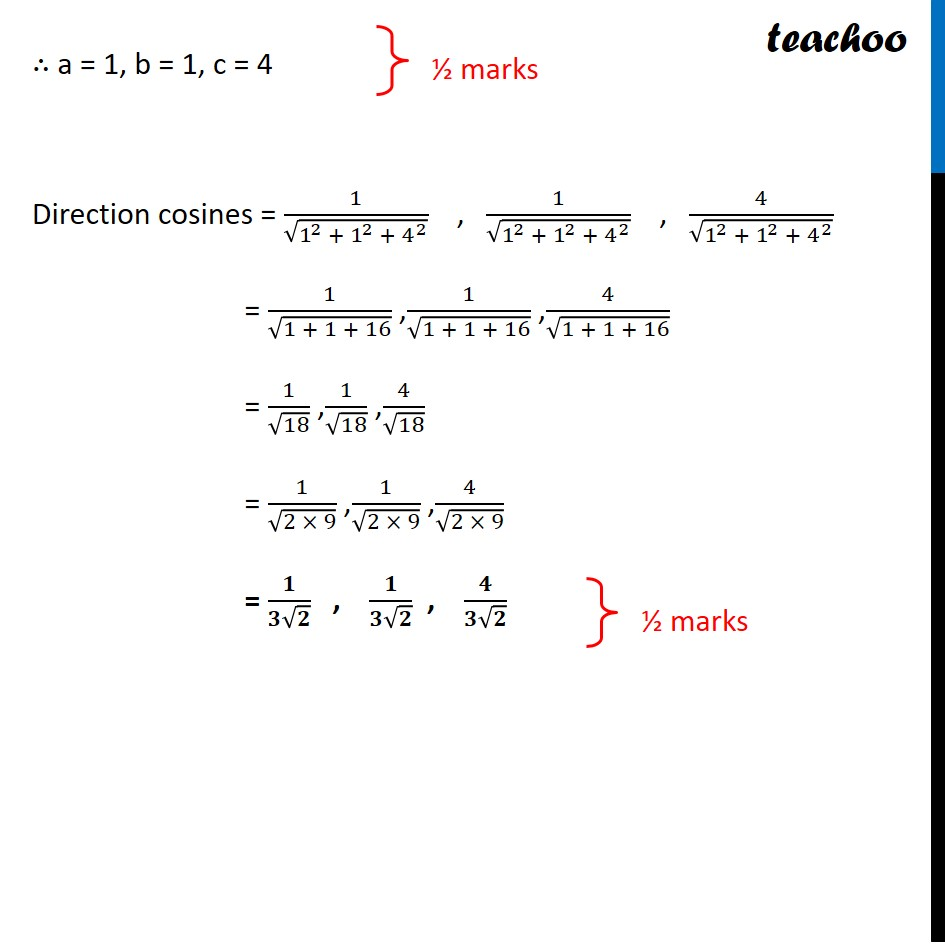CBSE Class 12 Sample Paper for 2022 Boards (For Term 2)

Class 12
Solutions of Sample Papers and Past Year Papers - for Class 12 Boards

## Find the direction cosines of the following line: (3 − 𝑥)/(−1) = (2𝑦 − 1)/2 = 𝑧/4

This question is similar to Question 4 or 2nd CBSE Class 12 Sample Paper 2019 BoardsLearn in your speed, with individual attention - Teachoo Maths 1-on-1 Class

### Transcript

Question 4 Find the direction cosines of the following line: (3 − 𝑥)/(−1) = (2𝑦 − 1)/2 = 𝑧/4 Given line (𝟑 − 𝒙)/(−𝟏) = (2𝑦 − 1)/2 = 𝑧/4 (−(𝑥 − 3) )/(−1) = (2𝑦 − 1)/2 = 𝑧/4 ((𝒙 − 𝟑) )/𝟏 = (2𝑦 − 1)/2 = 𝑧/4 ((𝑥 − 3) )/1 = 𝟐(𝒚 − ½)/𝟐 = 𝑧/4 ((𝑥 − 3) )/1 = ((𝒚 − ½))/𝟏 = 𝑧/4 So, direction ratios are 1, 1, 4 ½ marks ∴ a = 1, b = 1, c = 4 Direction cosines = 1/√(1^2 + 1^2 + 4^2 ) , 1/√(1^2 + 1^2 + 4^2 ) , 4/√(1^2 + 1^2 + 4^2 ) = 1/√(1 + 1 + 16) ,1/√(1 + 1 + 16) ,4/√(1 + 1 + 16) = 1/√18 ,1/√18 ,4/√18 = 1/√(2 × 9) ,1/√(2 × 9) ,4/√(2 × 9) = 𝟏/(𝟑√𝟐) , 𝟏/(𝟑√𝟐) , 𝟒/(𝟑√𝟐) ½ marks